# Math Help: Matrix Multiplication

08/14/2015

## Algebra Help: Multiplying Matrices

A matrix is a multi-dimensional group of numbers displayed as a rectangle instead of as a list, or vector. For example,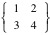is a matrix. If you have two matrices and want to multiply them together, you will end up with one answer matrix. To get there, you cannot simply start multiplying numbers, because multiplying matrices is not commutative. We will follow the complicated process of multiplying multi-dimensional matrices, using different numbers in each set to make it simpler to understand.

### Our Sample Matrices*### Step 1:

First add the products of the numbers in the top row of the first matrix multiplied by the numbers in the left column of the second matrix. In our example, we get 1*5=5 + 2*7=14 for a total of 19. This becomes the first number in our answer matrix.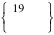### Step 2:

Now multiply the top row of the first matrix by the right column of the second matrix and add the products. We get 1*6=6 + 2*8=16 which totals 22.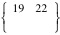### Step 3:

Repeat Steps 1 & 2, this time using the second row of the first matrix. 3*5=15 + 4*7=28 totals 43 and 3*6=18 + 4*8=32 totals 50. Our answer matrix looks like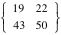and we have our answer. Just remember to multiply the rows of the first matrix by the columns in the second.

### More Than Two Dimensions

If your matrix is not a rectangle, but contains more dimensions to it, you need to add additional steps. To multiply matrices, the first matrix must have the same number of columns as the second matrix has rows. The rows in the first matrix and the columns in the second determine the size of the answer matrix. Let's look at an example.

### Multi-Dimensional Matrix Example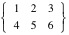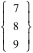### Step 1:

First make sure the columns and rows match up. The first matrix has three columns and the second matrix has three rows, so we may proceed. We portray the size of a matrix in the number of rows x the number of columns. The first matrix is 2x3 and the second is 3x1. The numbers in the middle must match up to multiply and the outer numbers give you the size of the answer matrix, 2x1 in this case.

### Step 2:

Multiply the rows of the first matrix by the columns of the second. 1*7, 2*8, 3*9 result in 7+16+27=50. Then 4*7, 5*8, 6*9 give us 28+40+56=124. Our answer matrix is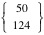.

## For Tutoring in Powder Springs, GA

Call The Tutoring Center in Powder Springs at 770-222-7133 to schedule a free diagnostic assessment for your child. We offer one-to-one tutoring help in math, reading, writing, and test preparations. For more information about our year-round academic programs online.

### FREE CONSULTATION

Schedule your Free Diagnostic Assessment Today!